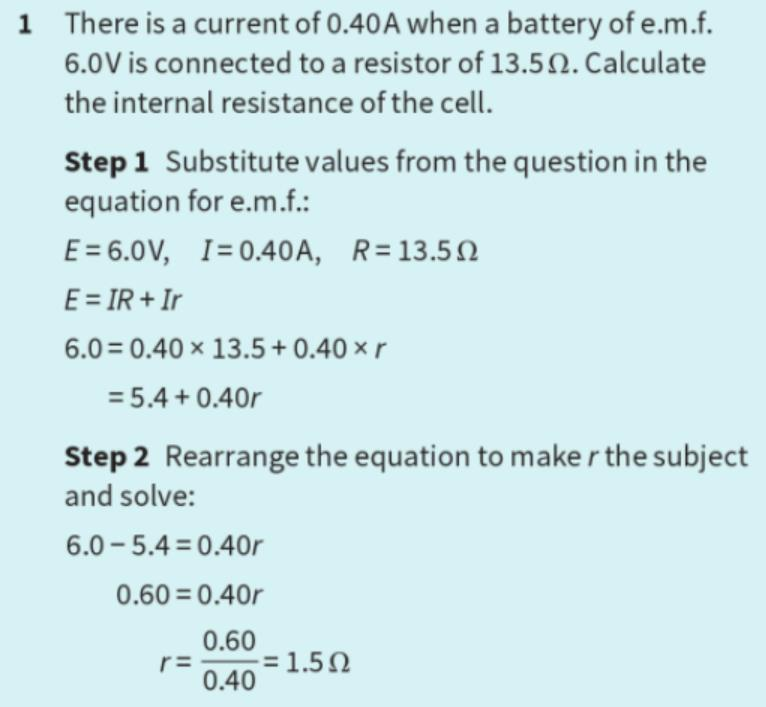Courses

# Test: Emfs & Internal Resistance

## 10 Questions MCQ Test Physics For JEE | Test: Emfs & Internal Resistance

Description
This mock test of Test: Emfs & Internal Resistance for JEE helps you for every JEE entrance exam. This contains 10 Multiple Choice Questions for JEE Test: Emfs & Internal Resistance (mcq) to study with solutions a complete question bank. The solved questions answers in this Test: Emfs & Internal Resistance quiz give you a good mix of easy questions and tough questions. JEE students definitely take this Test: Emfs & Internal Resistance exercise for a better result in the exam. You can find other Test: Emfs & Internal Resistance extra questions, long questions & short questions for JEE on EduRev as well by searching above.
QUESTION: 1

### A car battery has ________ resistance than a dry cell.

Solution:

a car battery of 12V provides a current of about 200A, which is the current needed to operate a starter motor. So a battery has a very small internal resistance.
also,The cause of non-correctable battery problems and.A weak cell holds less capacity and is discharged more quickly.

QUESTION: 2

Solution:
QUESTION: 3

### Electromotive force is a

Solution:

EMF is equal to potential difference between plates when the battery is not connected to any external circuit.
Potential difference is due to the charge accumulation on the plates. So, EMF produces a potential difference between the two terminals which drives the current.

QUESTION: 4

emf is measured in​

Solution:

The unit for electromotive force is Volt.
EMF is numerically expressed as the number of Joules of energy given by the source divided by each Coulomb to enable a unit electric charge to move across the circuit.
Volts=Joules/Coulombs

QUESTION: 5

Under what condition, the current drawn from the cell is maximum?​

Solution:

The maximum current can be drawn from a cell if the external resistance R = 0.

When current is to be drawn at max then the conducting wire rating comes high such that resistance is less.

Resistance R = rho x l/a

Rho = resistivity constant

L=length

a=area of cross-section

Hence, if the area of cross-section increases resistance decreases by increasing the rate of current to flow.

QUESTION: 6

Internal resistance of cell when there is current of 0.40 A when a battery of 6.0 V is connected to a resistor of 13.5 Ω is

Solution:QUESTION: 7

Two terminals of a cell of emf 2.5 V are connected to the two ends of the 10 ohm resistance coil. If the current in the circuit is 0.2 A, then the internal resistance of the cell is​

Solution:

The internal resistance of the cell can be obtained from the relation,
I=ε/R+r
0.2=2.5/(10+r)=2.5ohm

QUESTION: 8

Current provided by a battery is maximum when

Solution:

Current provided by a battery is maximum when internal resistance is equal to external resistance. As a battery discharges, not only does it diminish its internal store of energy, but its internal resistance also increases (as the electrolyte becomes less and less conductive), and its open-circuit cell voltage decreases (as the chemicals become more and more dilute). The most deceptive change that a discharging battery exhibits is increased resistance.

QUESTION: 9

What is the maximum current that can be drawn from a cell of emf E and internal resistance r connected to an external resistance R?

Solution:

as the maximum current that can be drawn from a cell is for R =0 therefore, it is I = E/r.

QUESTION: 10

The term open circuit means

Solution:

An open circuit implies that the two terminals are points that are externally disconnected, which is equivalent to a resistance R=∞ . This means that zero current can flow between the two terminals, regardless of any voltage difference.